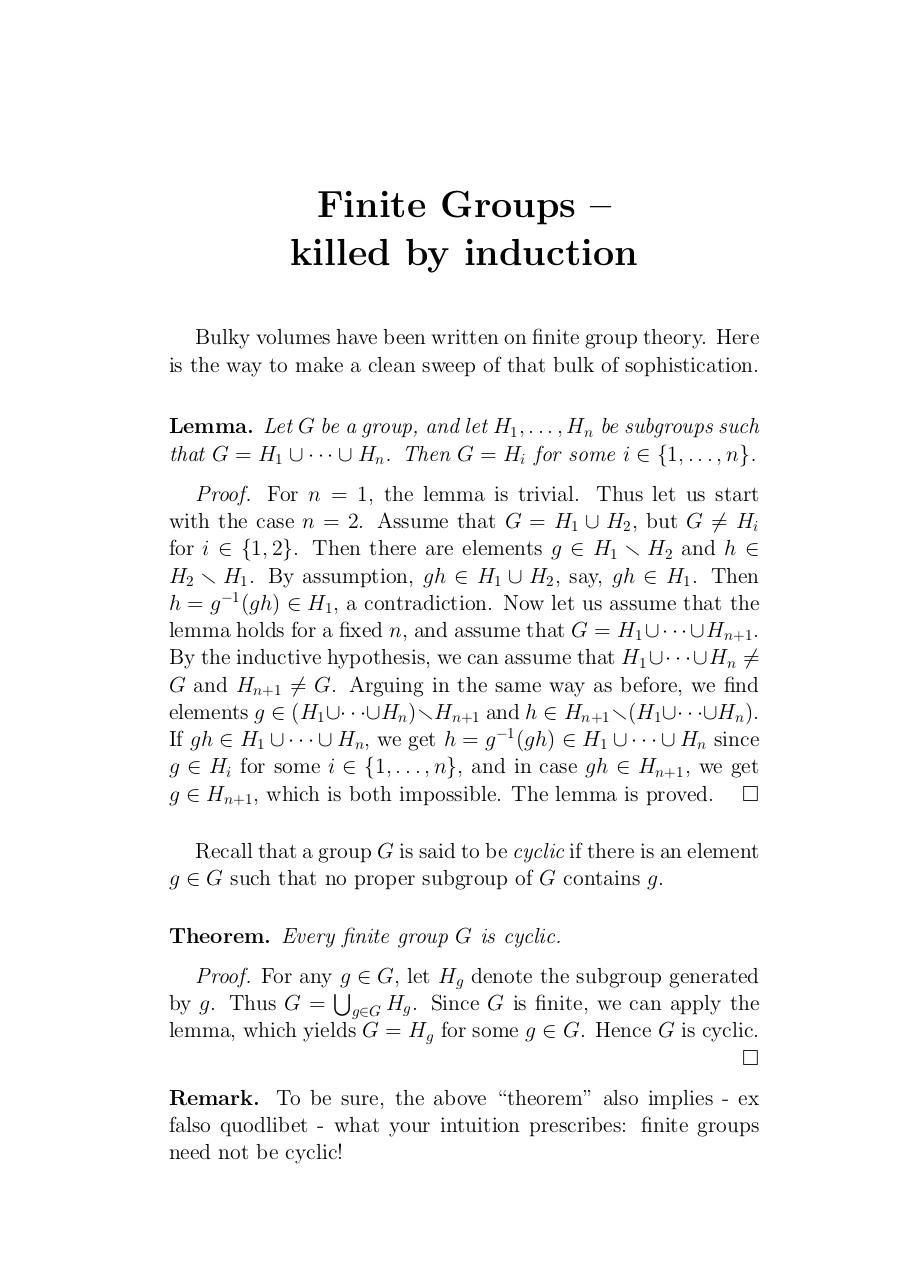# ind2 .pdf

### File information

Original filename: ind2.pdf

This PDF 1.3 document has been generated by / ESP Ghostscript 815.03, and has been sent on pdf-archive.com on 08/11/2015 at 15:34, from IP address 95.112.x.x. The current document download page has been viewed 488 times.
File size: 28 KB (1 page).
Privacy: public file

ind2.pdf (PDF, 28 KB)

### Document preview

Finite Groups –
killed by induction
Bulky volumes have been written on finite group theory. Here
is the way to make a clean sweep of that bulk of sophistication.
Lemma. Let G be a group, and let H1 , . . . , Hn be subgroups such
that G = H1 ∪ · · · ∪ Hn . Then G = Hi for some i ∈ {1, . . . , n}.
Proof. For n = 1, the lemma is trivial. Thus let us start
with the case n = 2. Assume that G = H1 ∪ H2 , but G 6= Hi
for i ∈ {1, 2}. Then there are elements g ∈ H1 r H2 and h ∈
H2 r H1 . By assumption, gh ∈ H1 ∪ H2 , say, gh ∈ H1 . Then
h = g −1 (gh) ∈ H1 , a contradiction. Now let us assume that the
lemma holds for a fixed n, and assume that G = H1 ∪ · · · ∪ Hn+1 .
By the inductive hypothesis, we can assume that H1 ∪· · ·∪Hn 6=
G and Hn+1 6= G. Arguing in the same way as before, we find
elements g ∈ (H1 ∪· · ·∪Hn )rHn+1 and h ∈ Hn+1 r(H1 ∪· · ·∪Hn ).
If gh ∈ H1 ∪ · · · ∪ Hn , we get h = g −1 (gh) ∈ H1 ∪ · · · ∪ Hn since
g ∈ Hi for some i ∈ {1, . . . , n}, and in case gh ∈ Hn+1 , we get
g ∈ Hn+1 , which is both impossible. The lemma is proved. 
Recall that a group G is said to be cyclic if there is an element
g ∈ G such that no proper subgroup of G contains g.
Theorem. Every finite group G is cyclic.
Proof. For any S
g ∈ G, let Hg denote the subgroup generated
by g. Thus G = g∈G Hg . Since G is finite, we can apply the
lemma, which yields G = Hg for some g ∈ G. Hence G is cyclic.

Remark. To be sure, the above “theorem” also implies - ex
falso quodlibet - what your intuition prescribes: finite groups
need not be cyclic!#### HTML Code

Copy the following HTML code to share your document on a Website or Blog

#### QR Code### Related keywords